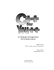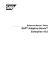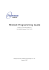# Exam Name___________________________________

## Transcription

Exam Name___________________________________
```Exam
Name___________________________________
MULTIPLE CHOICE. Choose the one alternative that best completes the statement or answers the question.
For the question(s) below, assume values is an int array that is currently filled to capacity, with the following values:
1) What is returned by values?
A) 9
B) 3
C) 2
D) 6
E) 12
2) What is the value of values.length?
A) 5
B) 18
C) 0
D) 7
E) 6
1)
2)
3) Which of the following loops would adequately add 1 to each element stored in values?
A) for(j=1;j<values.length-1;j++) values[j]++;
B) for(j=0;j<values.length;j++) values[j]++;
C) for(j=0;j<=values.length;j++) values[j]++;
D) for(j=0;j<values.length-1;j++) values[j]++;
E) for(j=1;j<values.length;j++) values[j]++;
3)
4) The statement System.out.println(values); will
A) cause a syntax error
B) output 7
C) cause an ArrayOutOfBoundsException to be thrown
D) output 18
E) output nothing
4)
5) Which of the following is a legal way to declare and instantiate an array of 10 Strings?
A) String s = new String;
B) String s = new String;
C) String[ ] s = new String;
D) String s = new String(10);
E) String[ ] s = new String;
5)
6) In Java, arrays are
A) objects
B) Strings
C) primitive data types if the type stored in the array is a primitive data type and objects if the
type stored in the array is an object
D) primitive data types
E) interfaces
6)
1
7) The "off-by-one" error associated with arrays arises because
A) the first array index is 0 and programmers may start at index 1, or may use a loop that goes
one index too far
B) the last array index is at length + 1 and loops may only iterate to length, missing one
C) programmers write a loop that goes from 0 to length - 1 whereas the array actually goes
from 1 to length
D) the last array element ends at length - 1 and loops may go one too far
E) none of the above, the "off-by-one" error has nothing to do with arrays
7)
8) What does the following code do? Assume list is an array of int values, temp is some previously
initialized int value, and c is an int initialized to 0.
for(j=0;j<list.length.j++)
if(list[j] < temp) c++;
A) It counts the number of elements in list that are less than temp
B) It sorts the values in list to be in ascending order
C) It finds the smallest value and stores it in temp
D) It counts the number of elements equal to the smallest value in list
E) It finds the largest value and stores it in temp
8)
An int array stores the following values. Use the array to answer the question(s) below.
9) Which of the following lists of numbers would accurately show the array after the first pass
through the Selection Sort algorithm?
A) 2, 4, 6, 8, 9, 12, 18
B) 2, 4, 9, 12, 6, 8, 18
C) 9, 4, 12, 2, 6, 8, 18
D) 4, 9, 12, 2, 6, 8, 18
E) 2, 4, 12, 9, 6, 8, 18
9)
10) Which of the following lists of numbers would accurately show the array after the second pass of
the Selection Sort algorithm?
A) 9, 4, 12, 2, 6, 8, 18
B) 2, 4, 6, 8, 9, 12, 18
C) 2, 4, 12, 6, 8, 9, 18
D) 2, 4, 12, 9, 6, 8, 18
E) 2, 4, 9, 6, 12, 8, 18
10)
11) Which of the following lists of numbers would accurately show the array after the fourth pass of
the Selection Sort algorithm?
A) 2, 4, 6, 9, 12, 8, 18
B) 9, 4, 12, 2, 6, 8, 18
C) 2, 4, 6, 9, 8, 12, 18
D) 2, 4, 6, 8, 12, 9, 18
E) 2, 4, 6, 8, 9, 12, 18
11)
12) How many passes will it take in all for Selection Sort to sort this array?
A) 5
B) 2
C) 7
D) 4
12)
2
E) 6
For the question(s) below, assume an int array, candy, stores the number of candy bars sold by a group of children where
candy[j] is the number of candy bars sold by child j. Assume there are 12 children in all.
13) What does the following code do?
int value1 = scan.nextInt( );
int value2 = scan.nextInt( );
bars[value1] += value2;
A) adds value1 to the number of bars sold by child value2
B) adds 1 to the number of bars sold by child value1 and child value2
C) adds value2 to the number of bars sold by child value1
D) inputs a new value for the number of bars sold by both child value1 and child value2
E) adds 1 to the number of bars sold by child value1
13)
14) Which of the following code could be used to compute the total number of bars sold by the
children?
A) for(int j=0; j<12; j++) sum+= candy[j];
B) for(int j=0; j<12; j++) candy[j] = sum;
C) for(int j=0; j<12; j++) sum = candy[j];
D) for(int j=0; j<12; j++) sum += [j];
E) for(int j=0; j<12; j++) [j] += sum;
14)
15) What does the following method do?
public int question15( )
{
int value1 = 0;int value2 = 0;
for(int j=0; j<12; j++)
if(candy[j] > value1)
{
value1 = candy[j];
value2 = j;
}
return value2;
}
A) It returns the total number of children who sold 0 candy bars
B) It returns the index of the child who sold the most candy bars
C) It returns the total number of candy bars sold
D) It returns the number of candy bars sold by the child who sold the most candy bars
E) It returns the total number of children who sold more than 0 candy bars
15)
16) We compare sorting algorithms by examining
A) the types of loops used in the sorting algorithm
B) the amount of memory space required by the algorithm
C) whether the resulting array is completely sorted or only partially sorted
D) the number of instructions executed by the sorting algorithm
E) the number of instructions in the algorithm itself (its length)
16)
3
17) Both the Insertion Sort and the Selection Sort algorithms have efficiencies on the order of ________
where n is the number of values in the array being sorted.
A) n 2
B) Insertion sort has an efficiency of n and Selection Sort has an efficiency of n2
C) n
D) n 3
E) n * log n
17)
18) Consider the array declaration and instantiation: int[ ] arr = new int; Which of the following is
A) It stores 5 elements with legal indices between 0 and 4
B) It stores 6 elements with legal indices between 0 and 5
C) It stores 5 elements with legal indices between 1 and 5
D) It stores 4 elements with legal indices between 1 and 4
E) It stores 5 elements with legal indices between 0 and 5
18)
19) If an int array is passed as a parameter to a method, which of the following would define the
parameter list for the method header?
A) (int[ ])
B) (int a[ ])
C) (a[ ])
D) (int a)
E) (int[ ] a)
19)
20) If int[ ] x = new int; and the statement x[-1] = 0; is executed, then which of the following
Exceptions is thrown?
A) NullPointerException
B) ArrayIndexOutOfBoundsException
C) NegativeArraySizeException
D) IndexOutOfBoundsException
E) ArithmeticException
20)
21) Assume that BankAccount is a predefined class and that the declaration BankAccount[ ]
firstEmpireBank; has already been performed. Then the following instruction reserves memory
space for
firstEmpireBank = new BankAccount;
A) 1000 reference variables and 1000 BankAccount entries
B) 1000 reference variables, each of which point to a single BankAccount entry
C) 1000 BankAccount entries
D) a reference variable to the memory that stores all 1000 BankAccount entries
E) a single BankAccount entry
21)
22) The following code accomplishes which of the tasks written below? Assume list is an int array that
stores positive int values only.
foo = 0;
for(j=0; j<list.length; j++)
if (list[j] > foo) foo = list[j];
A) it stores the largest value in list (the maximum) in foo
B) it stores the smallest value in list (the minimum) in foo
C) it counts the number of elements in list that are less than foo
D) it counts the number of elements in list that are greater than foo
E) it stores every value in list, one at a time, in foo, until the loop terminates
22)
4
23) If x is a char, and values is an int array, then values[x]
A) causes a syntax error
B) casts x as an int based on the digit that is stored in x (for instance, if x is '3' it uses 3) but
throws an exception if x does not store a digit
C) casts x as an int based on x's Unicode value (for instance, if x is 'a' then it uses 97 and if x is 'z'
then it uses 122)
D) casts x as an int based on x's position in the alphabet (for instance, if x is 'a' then it uses 0 and
if x is 'z' then it uses 25)
E) causes an Exception to be thrown
23)
24) To initialize a String array names to store the three Strings "Huey", "Duey" and "Louie", you would
do
A) String names = {"Huey", "Duey", "Louie"};
B) String[ ] names = {"Huey", "Duey", "Louie"};
C) String[ ] names = new String{"Huey", "Duey", "Louie"};
D) String names = {"Huey", "Duey", "Louie"};
E) String names; names = "Huey"; names = "Duey"; names = "Louie";
24)
25) To declare a two-dimensional int array called threeD, which of the following would you use?
A) int[ , ] twoD;
B) int twoD;
C) int [ [ ] ] twoD;
D) int[ ] twoD;
E) int[ ][ ] twoD;
25)
26) Which of the following statements loops through every element in the ArrayList alist?
A) for (alist : Object item)
B) for (Object item : alist)
C) for (Object alist : item)
D) for (Object item = null; item : alist)
E) for (item in alist)
26)
27) Which of the following correctly declares an ArrayList of Car objects?
A) ArrayList Car;
B) ArrayList cars = Car;
C) ArrayList[Car] cars;
D) ArrayList<Car> cars;
E) ArrayList Car[] cars;
27)
28) Which of the following expressions gives the last element in the ArrayList alist?
A) alist.get(alist.size())
B) alist.get(alist.last())
C) alist.last()
D) alist.get(alist.length())
E) alist.get(alist.size()-1)
28)
5
29) Which of the following statements adds item to the end of the ArrayList alist?
B) alist.set(alist.size()-1, item);
D) alist[alist.size()-1] = item;
E) both A and B
29)
For the question(s) below, use the following partial class definitions:
public class A1
{
public int x;
private int y;
public int z;
...
}
public class A2 extends A1
{
public int a;
private int b;
...
}
public class A3 extends A2
{
private int q;
...
}
30) Which of the following is true with respect to A1, A2 and A3?
A) A1 is a subclass of A2 and A2 is a subclass of A3
B) A3 is a subclass of A2 and A2 is a subclass of A1
C) A2 and A3 are both subclasses of A1
D) A1 and A2 are both subclasses of A3
E) A1, A2 and A3 are all subclasses of the class A
31) Which of the following lists of instance data are accessible in class A2?
A) a, b
B) x, y, z, a, b
C) x, y, z, a
D) z, a, b
32) Which of the following lists of instance data are accessible in A3?
A) a, q
B) x, y, z, a, b, q
C) x, z, a, q
D) x, a, q
E) a, b, q
6
30)
E) x, z, a, b
31)
32)
33) Which of the following is true regarding the use of instance variable y of class A1?
A) it is accessible in A1, A2 and A3
B) it is accessible in A1 and A2
C) it is accessible only in A3
D) it is not accessible to any of the three classes
E) it is accessible only in A1
33)
34) The instruction super( ); does which of the following?
A) calls the method super as defined in the current class
B) calls the method super as defined in the current class' parent class
C) calls the constructor as defined in the current class
D) calls the constructor as defined in the current class' parent class
E) calls the method super as defined in java.lang
34)
35) Inheritance through an extended (derirved) class supports which of the following concepts?
A) information hiding
B) correctness
C) modularity
D) code reuse
E) interfaces
35)
36) Which of the following is an example of multiple inheritance?
A) a computer can be a mainframe or a PC
B) a PC can be a desktop or a laptop
C) a laptop is both a PC and a portable device
D) a portable device is a lightweight device
E) Macintosh and IBM PC are both types of PCs
36)
37) Java does not support multiple inheritance, but some of the abilities of multiple inheritance are
available by
A) creating aliases
B) using public rather than protected or private modifiers
C) implementing interfaces
D) overriding parent class methods
E) importing classes
37)
38) All classes in Java are directly or indirectly subclasses of the ________ class.
A) Object
B) Reference
C) Wrapper
D) this
38)
39) Which of the following is not a method of the Object class?
A) equals
B) toString
C) hashCode
D) compareTo
E) all of the above are methods of the Object class
7
E) String
39)
40) Abstract methods are used when defining
A) derived classes
B) interface classes
C) classes that have no methods
D) classes that have no constructor
E) arrays
40)
8
For the question(s) below, consider the following class definition:
public class AClass
{
private int x;
private int y;
public AClass(int a, int b)
{
x = a;
y = b;
}
{
return x + y;
}
public void changeEm( )
{
x++;
y--;
}
public String toString( )
{
return "" + x + " " + y;
}
}
9
41) Consider that you want to extend AClass to BClass. BClass will have a third int instance variable,
z. Which of the following would best define BClass' constructor?
public BClass(int a, int b, int c)
A)
{
super(a, b, c);
}
public BClass(int a, int b, int c)
B)
{
x = a;
y = b;
z = c;
}
public BClass(int a, int b, int c)
C)
{
z = c;
}
public BClass(int a, int b, int c)
D)
{
super(a, b);
z = c;
}
public BClass(int a, int b, int c)
E)
{
super( );
}
41)
42) You want addEm to now add all three values and return the sum and changeEm to change x and
y, but leave z alone. Which should you do?
A) Redefine addEm to return the value of z + super.addEm( ), but leave changeEm alone
B) Redefine addEm and changeEm without referencing super.addEm( ) or super.changeEm( )
C) Redefine changeEm to call super.changeEm( ) and then set z = x + y, but leave addEm alone
D) Redefine addEm to return the value of z + super.addEm( ) and redefine changeEm to call
super.changeEm( ) and thenset z = x + y
E) Redefine changeEm to call super.changeEm( ) without doing anything to z, and redefine
42)
10
43) Which of the following would best redefine the toString method for BClass?
public String toString(int z)
A)
{
return " " + x + " " + y + " " + z;
}
public String toString( )
B)
{
return super.toString( );
}
public String toString( )
C)
{
return super.toString( ) + " " + z;
}
public String toString( )
D)
{
return super.toString( ) + " " x + " " + y + " " + z;
}
public String toString( )
E)
{
return " " + x + " + y + " " + z;
}
43)
44) Which of the following is true regarding Java classes?
A) All classes can have any number (0 or more) of parent classes and any number of children
(derived or extended) classes.
B) All classes must have 1 parent but may have any number of children (derived or extended)
classes.
C) All classes must have 1 parent class and may have a single child (derived or extended) class.
D) All classes must have 1 child (derived or extended) class but may have any number of parent
classes.
E) All classes can have either 0 or 1 parent class and any number of children (derived or
extended) classes.
44)
45) Two children of the same parent class are known as
A) brothers
B) relatives
C) clones
45)
D) aliases
E) siblings
46) A variable declared to be of one class can later reference an extended class of that class. This
variable is known as
A) public
B) derivable
C) polymorphic
D) cloneable
E) none of the above, a variable declared to be of one class can never reference any other type
of class, even an extended class
46)
47) In order to determine the type that a polymorphic variable refers to, the decision is made
A) by the user at run time
B) by the compiler at compile time
C) by the programmer at the time the program is written
D) by the operating system when the program is loaded into memory
E) by the Java run-time environment at run time
47)
11
For the question(s) below, assume that Student, Employee and Retired are all subclasses of Person, and all four classes have
different implementations of the method getMoney. Consider the following code where ... are the required parameters for the
constructors:
Person p = new Person(...);
int m1 = p.getMoney( );
// assignment 1
p = new Student(...);
int m2 = p.getMoney( );
// assignment 2
if (m2 < 100000) p = new Employee(...);
else if (m1 > 50000) p = new Retired(...);
int m3 = p.getMoney( );
// assignment 3
48) The reference to getMoney( ) in assignment 1 is to the class
A) Employee
B) Person
C) Student
D) Retired
E) this cannot be determined by examining the code
48)
49) The reference to getMoney( ) in assignment 2 is to the class
A) this cannot be determined by examining the code
B) Person
C) Retired
D) Student
E) Employee
49)
50) The reference to getMoney( ) in assignment 3 is to the class
A) Student
B) Retired
C) Employee
D) Person
E) this cannot be determined by examining the code
50)
51) The relationship between a child class and a parent class is referred to as a(n) ________
relationship.
A) has-a
B) instance-of
C) is-a
D) alias
E) was-a
51)
12
For the question(s) below, consider the following class definition:
public class Q
{
private int x;
public Q(int newValue)
{
x = newValue;
}
}
52) Which of the following is true about the class Q?
A) it's parent class is Java
B) it's parent class is Object
C) it cannot be extended
D) it has no parent class
E) it has a default child called Object
52)
53) If q1 and q2 are objects of Q, then q1.equals(q2)
A) throws a NullPointerException
B) is true if q1 and q2 reference the same Q object
C) is never true
D) is a syntax error since equals is not defined in the Q class
E) is true if q1 and q2 both store the same value of x
53)
54) A mouse event is a(n)
A) listener
B) GUI component
C) object
D) interface
E) all of the above
54)
For the question(s) below, use the following recursive method.
public int question1_2(int x, int y)
{
if (x == y) return 0;
else return question1_2(x-1, y) + 1;
}
55) If the method is called as question1_2(8, 3), what is returned?
A) 5
B) 24
C) 8
D) 11
E) 3
56) Calling this method will result in infinite recursion if which condition below is initially true?
A) (x < y)
B) (x != y)
C) (x = = 0 && y != 0)
D) (x > y)
E) (x = = y)
13
55)
56)
57) The following method should return true if the int parameter is even and either positive or 0, and
false otherwise. Which set of code should you use to replace ... so that the method works
appropriately?
public boolean question3(int x) { ... }
A) if (x = = 0) return false; else if (x < 0) return true; else return question3(x - 2);
B) if (x = = 0) return true; else if (x < 0) return false; else return question3(x - 2);
C) if (x = = 0) return true;
else if (x < 0) return false;
else return question3(x - 1);
D) return(x = = 0);
E) if (x = = 0) return false; else if (x < 0) return true; else return question3(x - 1);
57)
For the question(s) below, refer to the following recursive factorial method.
public int factorial(int x)
{
if (x > 1) return x * factorial (x - 1);
else return 1;
}
58) What is returned if factorial(3) is called?
A) 3
B) 1
C) 9
D) 0
E) 6
58)
59) What is returned if factorial(0) is called?
A) 1
B) nothing, factorial(0) causes infinite recursion
C) nothing, factorial(0) produces a run-time error
D) 0
E) 2
59)
60) How many times is the factorial method invoked if originally called with factorial(5)? Include the
original method call in your counting.
A) 6
B) 5
C) 4
D) 1
E) 7
60)
61) What condition defines the base case for this method?
A) (x <= 1)
B) (x <= 0)
C) (x = = 0)
61)
D) (x > 1)
E) (x = = 1)
62) What is wrong with the following recursive sum method? The method is supposed to sum up the
values between 1 and x (for instance, sum(5) should be 5 + 4 + 3 + 2 + 1 = 15).
public int sum(int x)
{
if(x = = 0) return 0;
else return sum(x - 1) + x;
}
A) the recursive case should return sum(x) + 1;
B) the method should return a boolean instead of an int
C) the base case should return 1 instead of 0
D) the base case condition should be (x <= 0) instead of (x = = 0)
E) the recursive case should return sum(x - 1) + 1; instead of sum(x - 1) + x;
14
62)
For the question(s) below, assume that int[ ] a = {6, 2, 4, 6, 2, 1, 6, 2, 5} and consider the two recursive methods below foo and
bar.
public int foo(int[ ] a, int b, int j)
{
if (j < a.length)
if (a[j] != b) return foo (a, b, j+1);
else return foo (a, b, j+1) + 1;
else return 0;
}
public int bar(int[ ] a, int j)
{
if (j < a.length)
return a[I] + bar(a, j+1);
else return 0;
}
63) What is the result of calling foo(a, 2, 0);?
A) 0
B) 1
C) 2
D) 3
E) 4
64) What is the result of calling foo(a, 3, 0);?
A) 0
B) 1
C) 2
D) 3
E) 4
65) What is the result of calling foo(a, 2, 9);?
A) 1
B) 0
C) 2
D) 3
E) 4
66) What is the result of calling bar(a, 0);?
A) 34
B) 5
C) 0
D) 6
E) 12
67) What is the result of calling bar(a, 8);?
A) 5
B) 0
C) 34
D) 12
E) 6
68) What does the following recursive method determine?
public boolean question16(int[ ]a, int[ ] b, int j)
{
if(j = = a.length) return false;
else if (j = = b.length) return true;
else return question16(a, b, j+1);
}
A) Returns true if a and b are equal in size, false otherwise
B) Returns true if b is larger than a, false otherwise
C) Returns true if a is larger than b, false otherwise
D) Returns true if a and b have no elements
E) Returns the length of array a + length of array b
15
63)
64)
65)
66)
67)
68)
69) Why is the following method one which has infinite recursion?
public int infiniteRecursion(int n)
{
if (n > 0) return infiniteRecursion(n) + 1;
else return 0;
}
A) Because the base case will never be true
B) Because the recursive call does not move the parameter closer to the base case
C) Because there is no base case
D) Because the recursive call moves the problem further away from the base case
E) none of the above, there is no infinite recursion in this method
69)
For the question(s) below, recall the Towers of Hanoi recursive solution.
70) If there are 2 disks to move from one Tower to another, how many disk movements would it take
to solve the problem using the recursive solution?
A) 0
B) 1
C) 2
D) 3
E) 4
70)
71) If there are 6 disks to move from one Tower to another, how many disk movements would it take
to solve the problem using the recursive solution?
A) 6
B) 13
C) 31
D) 127
E) 63
71)
72) The solution to the Towers of Hanoi has a(n) ________ complexity.
A) polynomial
B) logarithmic
C) linear
D) exponential
72)
16
For the question(s) below, consider the following representation of grid and the maze code from Chapter 8.
Grid:
11111100
00100100
00100110
00110010
00011000
00001111
Code:
public boolean traverse(int row, int column)
{
if (valid(row, column))
{
boolean done = false;
grid[row][column] = TRIED;
if (row == grid.length - 1 && column == grid.length - 1)
done = true;
else
{
done = traverse(row + 1, column);
if(!done) done = traverse(row, column + 1);
if(!done) done = traverse(row - 1, column);
if(!done) done = traverse(row, column - 1);
}
if(done) grid[row][column] = PATH;
}
return done;
}
Assume valid returns true if row and column are >= 0 and <= the grid's row length or column length and the entry at this
position = = 1. And assume TRIED = 3 and PATH = 7
73) If traverse is first called with traverse(0, 0); what will the first recursive call of traverse be?
A) traverse(0, -1);
B) traverse(1, 1);
C) traverse(0, 1);
D) traverse(0, 0);
E) traverse(1, 0);
73)
74) Assume at some point in processing, grid's row 0 has become 3 3 3 1 1 1 0 0. Which direction will
next be tried?
A) left
B) up
C) down
D) right
E) none, the recursion ends at this point
74)
17
75) Which of the following grids would be the result after traverse has completed all of its recursive
calls?
77733300
A)
00700300
00700330
00770030
00077000
00007777
11111100
B)
00100100
00100110
00110010
00011000
00001111
33377700
C)
00300700
00300770
00330070
00033000
00003333
33333300
D)
00300300
00300330
00330030
00033000
00003333
77777700
E)
00300700
00300770
00330070
00033000
00003333
75)
76) Define the magnitude of a number as the location of the decimal point from the left of the number
(that is, if a number has 4 digits followed by the decimal point, it will have a magnitude of 4). 100
would then have a magnitude of 3 and 55,555.555 would have a magnitude of 5. A partial
recursive method is given below to compute a positive int parameter's magnitude. Which answer
below is needed to complete the method?
public int magnitude(double x)
{
if (x < 1) return 0;
else return ________;
}
A) magnitude(x / 10) + 1;
B) magnitude(x / 10) + 10;
C) magnitude(x - 1) + 10;
D) magnitude(x / 10) * 2;
E) magnitude(x - 10) + 1;
76)
18
The following method recognizes whether a String parameter consists of a specific pattern and returns true if the String has
that pattern, false otherwise. Use this recursive method to answer the question(s) below.
public boolean patternRecognizer(String a)
{
if(a == null) return false;
else if (a.length( ) = = 1 | | (a.length( ) = = 2 && a.charAt(0) = =
a.charAt(1) ) ) return true;
else if (a.length( ) = = 2 && a.charAt(0) != a.charAt(1) ) return false;
else if (a.charAt(0) == a.charAt(a.length( ) - 1))
return patternRecognizer(a.substring(1, a.length( ) - 1));
else return false;
}
77) Which String below would result in patternRecognizer returning true?
A) "abcba"
B) "aabba"
C) "aaabbb"
D) "abcde"
E) all of the above Strings will result in the method returning true
77)
78) If the method is called as patternRecognizer(x) where x is "aa", what will the result be?
A) true
B) infinite recursion
C) false
D) a NullPointerException
E) a run-time error
78)
79) If the statement a.substring(1, a.length( ) - 1) were changed to be (a.substring(1, a.length( )), then
the method would
A) always return true
B) return true only if all characters of the String were the same
C) have infinite recursion unless the String were null
D) return true only the String had only two characters and they were the same
E) always return false
79)
19
Testname: AP CHAPTER 6 7 8
1)
2)
3)
4)
5)
6)
7)
8)
9)
10)
11)
12)
13)
14)
15)
16)
17)
18)
19)
20)
21)
22)
23)
24)
25)
26)
27)
28)
29)
30)
31)
32)
33)
34)
35)
36)
37)
38)
39)
40)
41)
42)
43)
44)
45)
46)
47)
48)
49)
50)
C
D
B
C
C
A
A
A
E
D
D
E
C
A
B
D
A
A
E
B
B
A
C
B
E
B
D
E
A
B
E
C
E
D
D
C
C
A
D
B
D
A
C
B
C
C
E
B
D
E
20
Testname: AP CHAPTER 6 7 8
51)
52)
53)
54)
55)
56)
57)
58)
59)
60)
61)
62)
63)
64)
65)
66)
67)
68)
69)
70)
71)
72)
73)
74)
75)
76)
77)
78)
79)
C
B
B
C
A
A
B
E
A
B
A
D
C
A
B
A
A
C
B
D
E
D
E
D
A
A
A
A
B
21
```

### IRC channels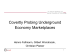### Membership Package - Golden Eagle Golf Club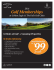### 9. Abstract Data Types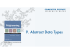### Shortest Paths - Computer Science Department at Princeton University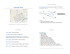### 15 April 2016 - Tawa Intermediate School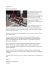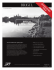### KS0108 Graphic LCD Library For AVR Microcontrollers GCC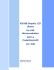### Absolute Unreserved Auction### cpik C compiler for pic 18Fxxx - PiKdev, An IDE for the development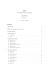### Embedded Systems Design Course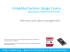### C++ Quick Syntax Reference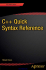### May 2014 - SHARE Foundation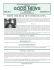### ebook - The C Progra.. - UTN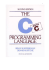### asymptote.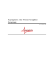### \$11.95 CAN \$ 8.95 US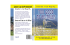### C-Programming for ECE Majors Text Book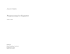### CipherLab C Programming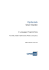### Programming with lcc-win32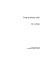### Programming with lcc-win - BYU Computer Science Students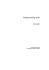### C++ for You++ - Skylight Publishing